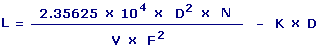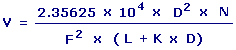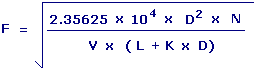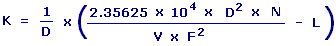Subwoofer Vent Length Calculators Enter value, select unit and click on calculate. Result will be displayed.

Calculate Length of Vent or Port
Calculate Volume of Box
Calculate Tunning Frequency
Calculate End Correction Factor

 Web www.calculatoredge.com

Calculate Length of Vent or PortL = Length of Vent or Port D = Diameter of Vent N = Number of Ports V = Volume of Box F = Tuning Frequency K = End Correction Factor
 Enter your values: Diameter of Vent: Centimeter Meter Attometer Dekameter Decimeter Exameter Femtometer Foot Hectometer Inch Kilometer Megameter Micrometer Mile Millimeter Nanometer Petameter Picometer Yard Number of Ports: Volume of Box: Liter Meter^3 Centimeter^3 Deciliter Foot^3 Inch^3 Kilometer^3 Milliliter Yard^3 Cord Firewood Cord Foot Wood Gallon UK Gallon US Liquid Ounce UK Fluid Ounce US Fluid Quart US Tuning Frequency: Hertz 1 / Second Cycle / Second Rotation / Second End Correction Factor: Result: Vent Length: Centimeter

Calculate Volume of BoxV = Volume of Box D = Diameter of Vent N = Number of Ports F = Tuning Frequency L = Length of Vent or Port K = End Correction Factor
 Enter your values: Diameter of Vent: Centimeter Meter Attometer Dekameter Decimeter Exameter Femtometer Foot Hectometer Inch Kilometer Megameter Micrometer Mile Millimeter Nanometer Petameter Picometer Yard Number of Ports or Vents: Tuning Frequency: Hertz 1 / Second Cycle / Second Rotation / Second Vent Length: Centimeter Meter Attometer Dekameter Decimeter Exameter Femtometer Foot Hectometer Inch Kilometer Megameter Micrometer Mile Millimeter Nanometer Petameter Picometer Yard End Correction Factor: Result: Volume of Box: Liter

Calculate Tuning FrequencyF = Tuning Frequency D = Diameter of Vent N = Number of Ports V = Volume of Box L = Length of Vent or Port K = End Correction Factor
 Enter your values: Diameter of Vent: Centimeter Meter Attometer Dekameter Decimeter Exameter Femtometer Foot Hectometer Inch Kilometer Megameter Micrometer Mile Millimeter Nanometer Petameter Picometer Yard Number of Ports or Vents: Volume of Box: Liter Meter^3 Centimeter^3 Deciliter Foot^3 Inch^3 Kilometer^3 Milliliter Yard^3 Cord Firewood Cord Foot Wood Gallon UK Gallon US Liquid Ounce UK Fluid Ounce US Fluid Quart US Vent Length: Centimeter Meter Attometer Dekameter Decimeter Exameter Femtometer Foot Hectometer Inch Kilometer Megameter Micrometer Mile Millimeter Nanometer Petameter Picometer Yard End Correction Factor: Result: Tuning Frequency: Hertz

Calculate End Correction FactorK = End Correction Factor D = Diameter of Vent N = Number of Ports L = Length of Vent or Port F = Tuning Frequency V = Volume of Box
 Enter your values: Diameter of Vent: Centimeter Meter Attometer Dekameter Decimeter Exameter Femtometer Foot Hectometer Inch Kilometer Megameter Micrometer Mile Millimeter Nanometer Petameter Picometer Yard Number of Ports or Vents: Volume of Box: Liter Meter^3 Centimeter^3 Deciliter Foot^3 Inch^3 Kilometer^3 Milliliter Yard^3 Cord Firewood Cord Foot Wood Gallon UK Gallon US Liquid Ounce UK Fluid Ounce US Fluid Quart US Tuning Frequency: Hertz 1 / Second Cycle / Second Rotation / Second Vent Length: Centimeter Meter Attometer Dekameter Decimeter Exameter Femtometer Foot Hectometer Inch Kilometer Megameter Micrometer Mile Millimeter Nanometer Petameter Picometer Yard Result: End Correction Factor:LINKSDISCLAIMERCONTACT US